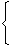# Exam Mission

Exam Information| Govt Jobs.| Exam Preparation | Exam Study Material |Exam Date|Material PDF

1. 'BODMAS' Rule:
This rule depicts the correct sequence in which the operations are to be executed, so as to find out the value of given expression.
Here B - Bracket,
O - of,
D - Division,
M - Multiplication,
S - Subtraction
Thus, in simplifying an expression, first of all the brackets must be removed, strictly in the order (), {} and ||.
After removing the brackets, we must use the following operations strictly in the order:
(i) of (ii) Division (iii) Multiplication (iv) Addition (v) Subtraction.
2. Modulus of a Real Number:
Modulus of a real number a is defined as
 |a| =a, if a > 0 -a, if a < 0
Thus, |5| = 5 and |-5| = -(-5) = 5.
3. Virnaculum (or Bar):
When an expression contains Virnaculum, before applying the 'BODMAS' rule, we simplify the expression under the Virnaculum.